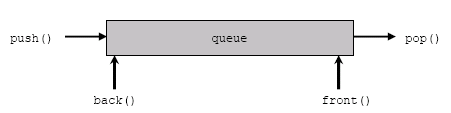×

Search anything:

# Different ways to initialize a queue in C++ STL

#### Software Engineering C++In this article, we have explored different ways to initialize a queue in C++ Standard Template Library (STL). There are three ways to initialize:

1. Member functions for inserting elements
2. Container objects
3. Another Queue

Before moving into the details of initialization, we will go through the basic knowledge of queue in C++ STL.

A Queue is a data structure that implements the First In First Out(FIFO) principle i.e the first element going into the queue is the first element to be taken out. You can visualize it exactly like a 'disciplined' queue in a market. A queue has 2 ends: front and back. Elements to be added to the queue are inserted from the back of the queue whereas elements are removed from the front of the queue.
Such is the structure of a queue:The C++ STL provides 10 Container classes that have member functions that can be used to manipulate the data.

Types of Containers are:

1. First-class Containers/Sequence Containers: Include vector, deque and list
2. Associative Containers : Include set, map, Multiset and Multimap
3. Container adaptors/Derived Containers : Include Queue, Stack, Priority queue

All Container adaptors internally use suitably modified first-class Containers such that they achieve the desired functionality.

# Introduction

Queue is a Container adaptor that can be used whenever a standard queue is required in the program. It does not have any special iterators of it's own(Iterators are components of STL, used to traverse Containers). It can use the first class container objects for its initialization. A queue is declared as follows:

``````queue<int> q;    // template<class T> identifier;
``````

During declaration, the base container of the queue can also be explicitly mentioned: template<class T,class Container> identifier
Generally, a queue implements deque as its default base container.

``````queue<int , deque<int> > q1;
``````

However, one can easily change the base container by simply mentioning the name of the desired base container in the declaration of the queue. Just like this:

``````queue<int , list<int> >q2;
``````

The above mentioned two examples create an empty queue with deque and list as the base container, respectively.

A queue can be initialized to the values of an existing list or deque.
Queue cannot be initialized to vectors as the vector class does not support the pop() function and hence removing elements from the queue is not possible which hinders its functionality and dissolves its purpose.

## Queue can be initialized using:

1. Member functions for inserting elements
2. Container objects
3. Another Queue

Let's discuss each of them :

# 1.Inserting elements using member functions

This is the most trivial method for initializing a queue.Elements are simply inserted into the queue using the push() member function. Another member function called emplace() can also be used. Just like push(),emplace() inserts elements at the end of the queue. Following is an example :

``````#include<iostream>
#include<queue>
using namespace std;
int main()
{
queue<int> q;
q.push(1);
q.push(4);
q.push(9);
q.push(16);
q.push(25);
while(!q.empty())
{
cout<<q.front()<<" ";
q.pop();
}
return 0;
}
``````

Output

``````1 4 9 16 25
``````

# 2. Container objects

Objects of first-class Containers like deque and list are used to initialize a queue.

Example

``````#include <iostream>
#include <queue>
#include <deque>
using namespace std;
int main(){
deque<int> dq ;
for(int i=1;i<=5;i++)
dq.push_back(i);      //inserting 5 elements in the deque
queue<int> q(dq);           //initializing q using dq
q.push(10);
q.pop();
while(!q.empty()){
cout<<q.front()<<" ";
q.pop();
}

return 0;
}
``````

Output

``````2 3 4 5 10
``````

This example initializes a queue (q) with a deque (dq) containing 5 elements.
Similarly,a queue can be initialized using a list. Here, a queue (q) is initialized using a list (lt).

Example

``````#include <iostream>
#include <list>
#include <queue>
using namespace std;
int main()
{
list<int> lt(5,10); // initializing a list with 5 elements
queue<int,list<int> > q(lt);   // q is initialized to the values of the list
while(!q.empty())
{
cout<<q.front()<<" ";
q.pop();
}
return 0;
}
``````

Output

``````10 10 10 10 10
``````

# 3. Another Queue

Another queue of the same data type and base container can be used to initialize a queue. Simply pass the queue, just like a constructor parameter.

Example

``````#include <iostream>
#include <queue>
using namespace std;

int main()
{
queue<int> past;
past.push(10);
past.push(20);
past.push(30);
queue<int> present(past); //present is initialized to past
while(!present.empty()){
cout<<present.front()<<" ";
present.pop();
}
return 0;
}
``````

Output

``````10 20 30
``````

## Question

#### Which one of the following is not a member function of the queue STL class?

get(int i)
swap()
emplace()
back()
get(int) is used for accessing elements in Hashmaps,Arraylists in java etc.
All other options are member functions of the Queue container in c++ stl.
1) swap() is used to swap elements of 2 queues
2) emplace() is used to insert elements at the end of the queue
3) back() is used to get a reference to the last element in the queue